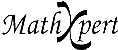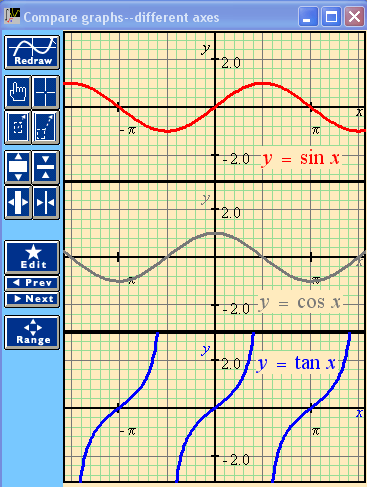# Help With Math: Purveyors of MathXpert

-->This type of graph allows the student to compare functions on different axes, lined up vertically. This example compares the three most basic trigonometric functions. Can you see from the graphs how it makes sense that tan x = (sin x) / cos x?